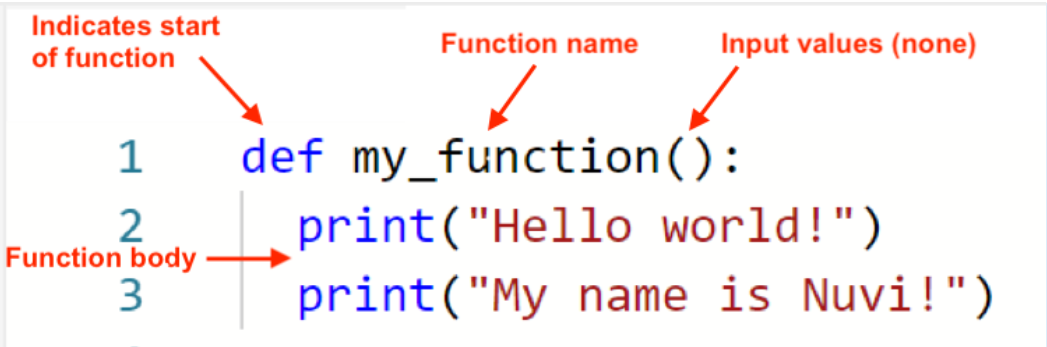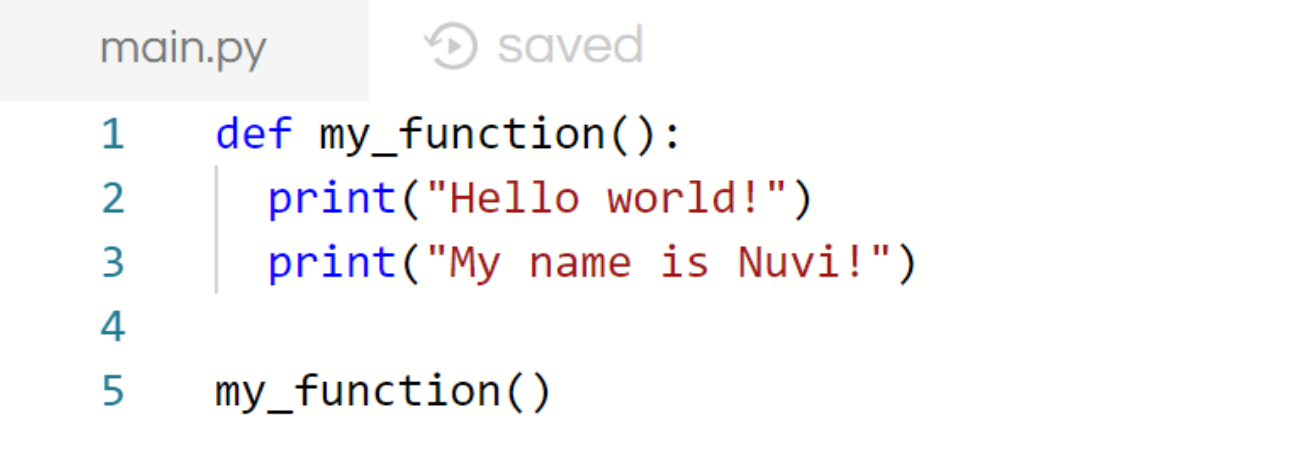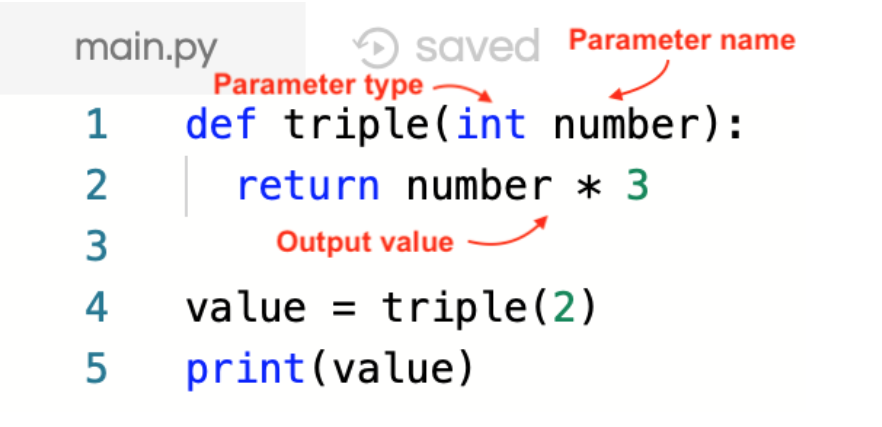Suppose you have many lines of code, and you would like to give this section of code a meaningful name. Functions are blocks of code that do just that. A function is a set of instructions that we give to the computer to store, and it can take in parameters (input data) and output data. A function in Python looks like this:Let’s analyze this piece of code. The def tells the computer that you are writing a function. We give it a name, in this case, my_function. Then, we put a colon (the : symbol) to indicate that the function is starting. The next two lines are two spaces away from the left side of the text editor – this tells the computer that these lines are part of the function definition. This is very important, so don’t miss out on this detail!

Press run.

You will notice that nothing happens. This is only a function definition - we need to “call” the function to run the code within the function. In other words, the computer understands the instructions, but you need you tell the computer to do the given instructions. You can call my_function() anywhere, as long as it is after the function is defined. Place my_function() below the function definition, with no spaces in front. Your code should look like this:You might be familiar with the math definition of a function; for example, the function 𝒇(𝒙) = 3𝒙. It takes an input value, 𝒙, and transforms the value by multiplying it by 3. Similarly, in python, you can also give input values (called parameters) to a function and return (or produce) an output value. An example of a Python function which triples a given number is given below:Number is the name of the parameter (input) that we are giving to the function triple. The word int tells the computer that the parameter number is an integer. The return word is only used within functions, and allows the function to output a value. In this case the triple function returns (outputs) number * 3, which then can be stored in a variable and printed. If the function variable. Check that the code above prints out the number 6.